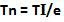## Wednesday, January 6, 2010

### Irodov Problem 1.376

The number of particles impinging upon the target each second is given byThe momentum of each particle is given by the solution in Problem 1.375 as,The total change in momentum of all the n particles each second is the force imparted in the target and is given by,The total energy of the n particles getting absorbed into the target is